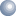## What is Power Factor?

### Power Factor Definition:

Power factor is the ratio between the kW and the kVA drawn by an electrical load where the kW is the actual load power and the kVA is the apparent load power. It is a measure of how efficiently the current is being converted into useful work output and more particularly is a good indicator of the effect of the load current on the efficiency of the supply system.

### What causes Power Factor to change?

All current drawn in a circuit will cause losses in the supply system. A load with a power factor of unity or 1.0 is the most efficient loading of the supply and a load with a PF of 0.7 or lower increases the losses in the system. A poor power factor is the result of a significant phase difference between the voltage and current caused by the load on the supply, or it can be due to a high harmonic content or a distorted waveform. Poor load current phase angle is generally the result of inductive loads such as lightly loaded electric motors, power transformers, fluorescent or high bay lighting ballasts and welding sets. A distorted current waveform may be caused by variable speed drives, switched mode power supplies, discharge lighting, rectifiers, or other electronic type loads.
In 3 phase power supplies the "power" can be measured as a triangle.ACTIVE Power is the base line and is the real usable power measured in kW. REACTIVE power is the vertical or that part of the supply which causes the inductive load. The reactive power in is measured in kVAr (kilo volt-amperes reactive) APPARENT Power is the hypotenuse. This is the resultant of the other two components and is measured in kVA
Power Factor is expressed as COS phi Ø (The angle between Apparent Power and Active power)

How does Power Factor Correction work?
Capacitive Power Factor correction (PFC) is applied to electric circuits as a means of minimising the inductive component of the current and thereby reducing the losses in the supply. The introduction of Power Factor Correction capacitors is a widely recognised method of reducing an electrical load, thus minimising wasted energy and hence improving the efficiency of a plant and reducing the electricity bill. It is not usually necessary to reach unity, ie Power Factor 1, since most supply companies are happy with a PF of 0.95 to 0.98 By installing suitably sized and controlled switched banks of capacitors into the circuit, the Power Factor is improved and the value becomes nearer to 1 thus minimising wasted energy and improving the efficiency of a plant.

The capital cost of the installation is usually repaid in less than 1 years electricity savings.This document maintained by ajmenergy@btinternet.com.
.